# RD Sharma Solutions for Class 9 Maths Chapter 15 Area of Parallelograms and Triangles Exercise VSAQs

Get free RD Sharma Solutions for Class 9 Maths Chapter 15 exercise VSAQs here. This exercise helps students to revise all the concepts they studied in this chapter. Students can download RD sharma class 9 solutions for exercise VSAQs and clear their doubts. All questions are solved by subject experts in accordance with CBSE guidelines. This study material is designed in simple language so that each concept can be easily grasped by the students.

## Download PDF of RD Sharma Solutions for Class 9 Maths Chapter 15 Area of Parallelograms and Triangles Exercise VSAQs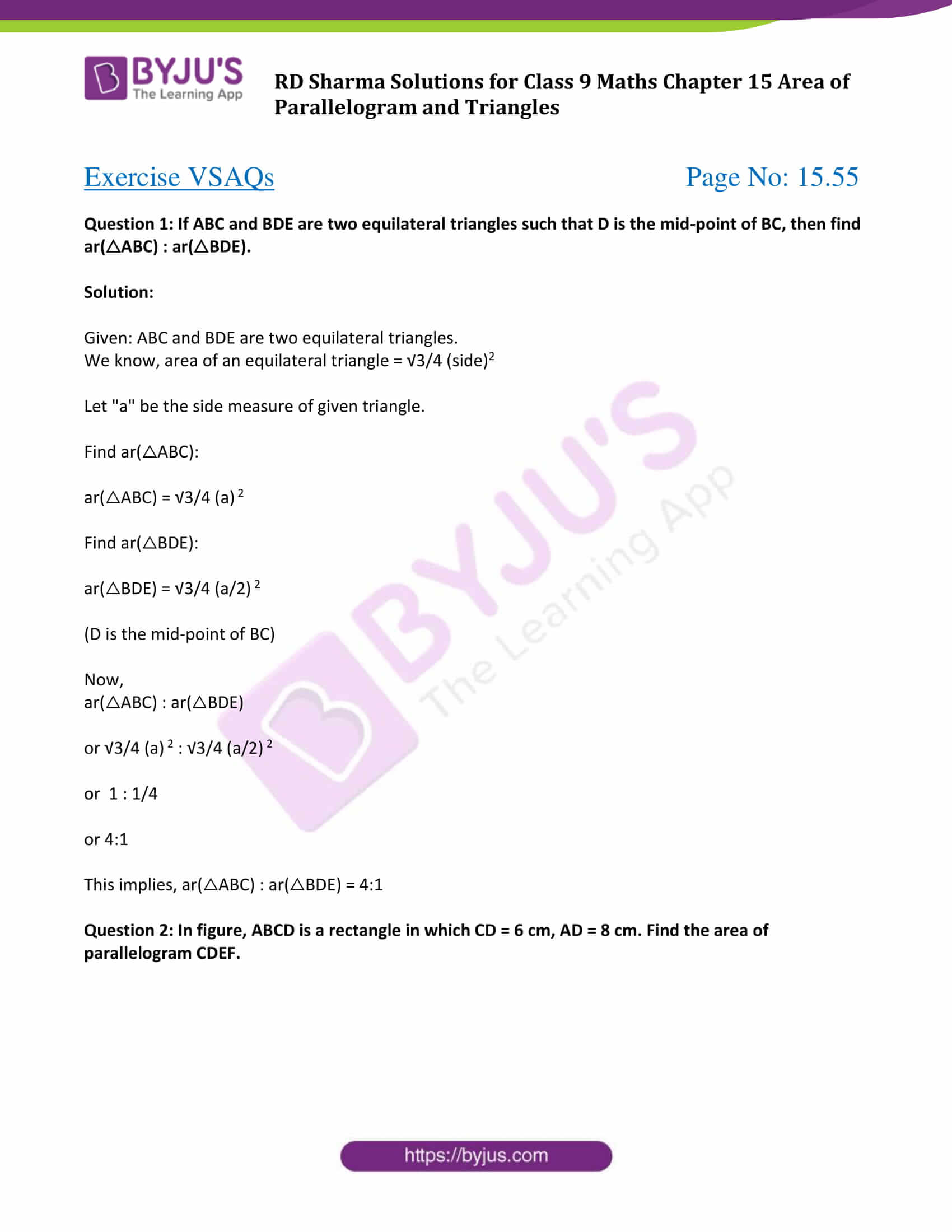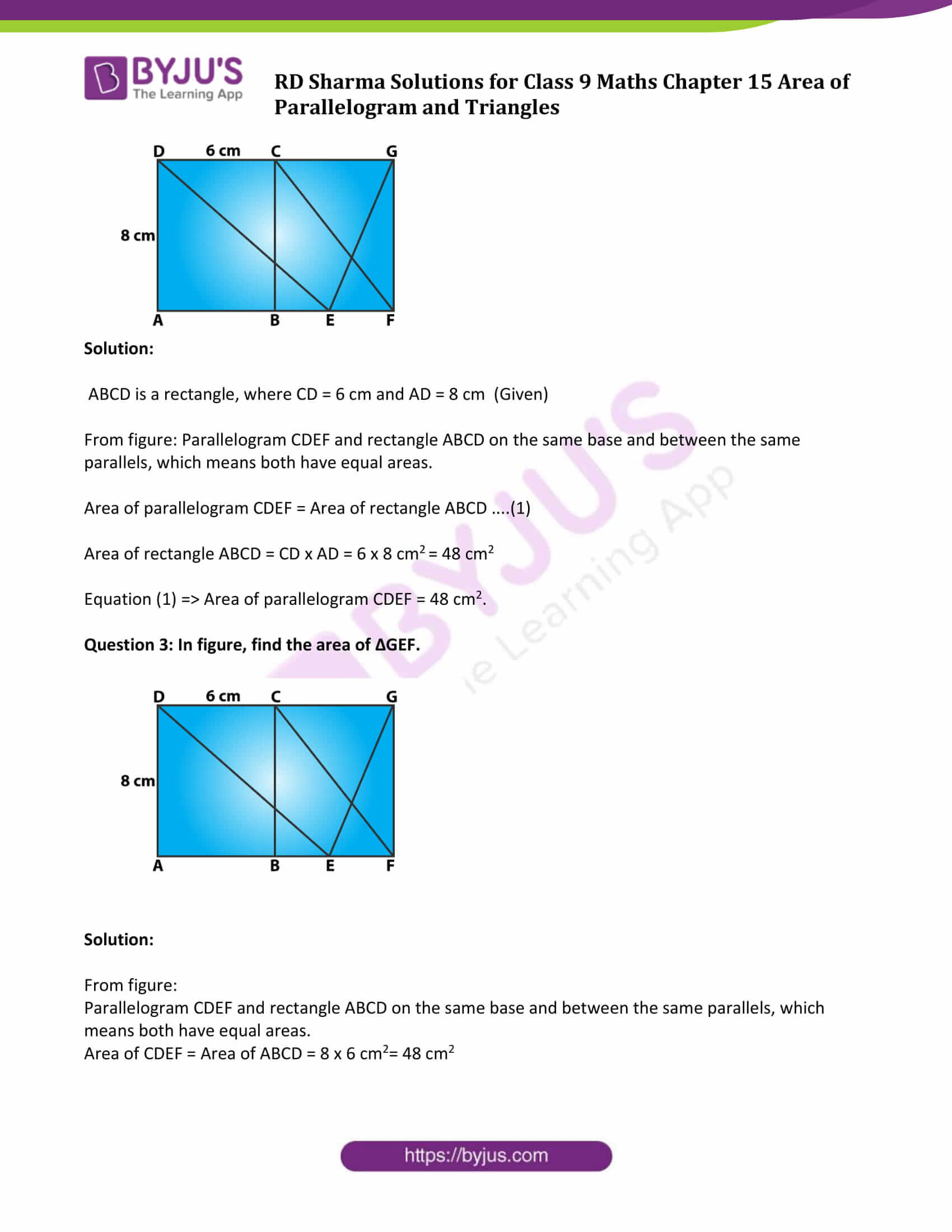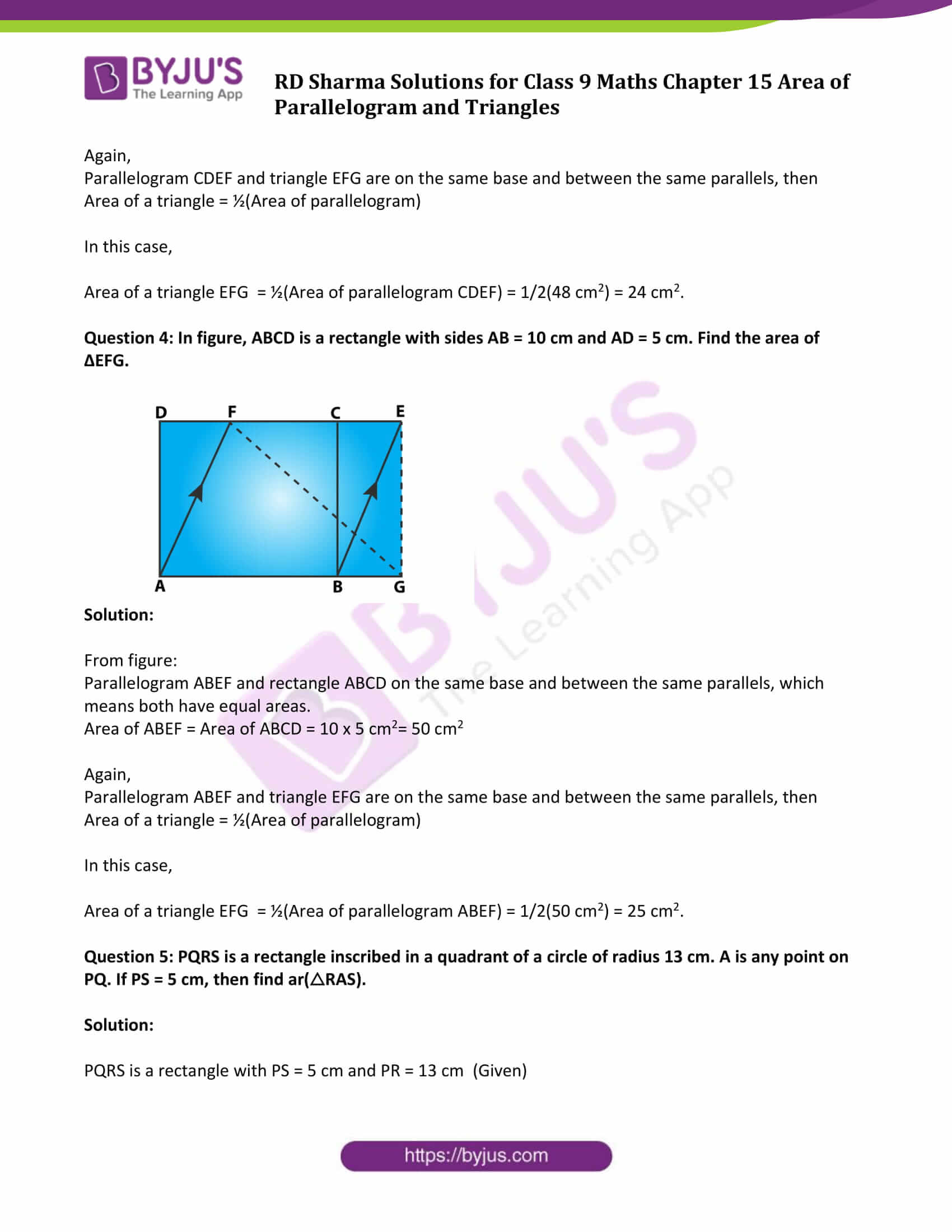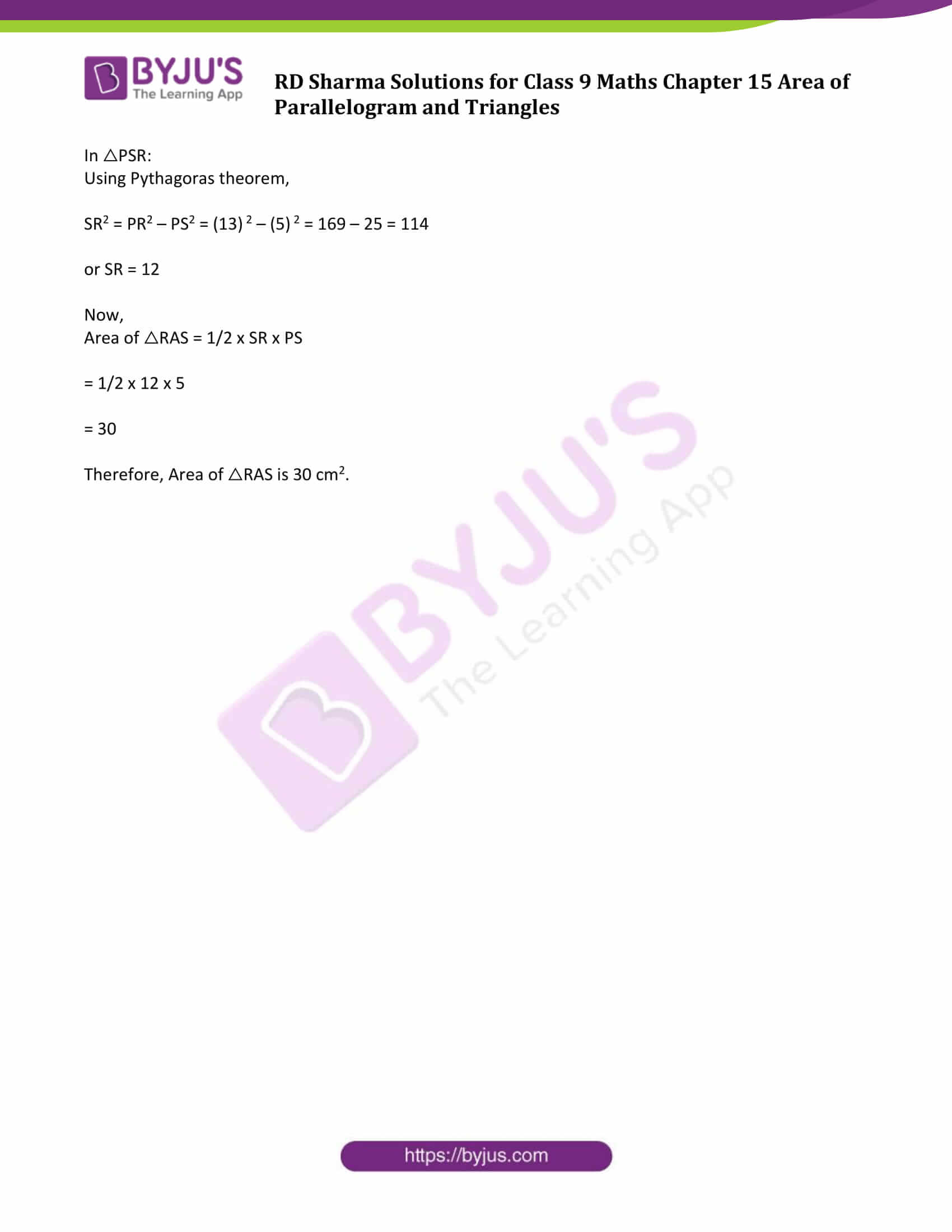### Access Answers to Maths RD Sharma Solutions for Class 9 Chapter 15 Area of Parallelograms and Triangles Exercise VSAQs Page number 15.55

Question 1: If ABC and BDE are two equilateral triangles such that D is the mid-point of BC, then find ar(△ABC) : ar(△BDE).

Solution:

Given: ABC and BDE are two equilateral triangles.

We know, area of an equilateral triangle = √3/4 (side)2

Let “a” be the side measure of given triangle.

Find ar(△ABC):

ar(△ABC) = √3/4 (a) 2

Find ar(△BDE):

ar(△BDE) = √3/4 (a/2) 2

(D is the mid-point of BC)

Now,

ar(△ABC) : ar(△BDE)

or √3/4 (a) 2 : √3/4 (a/2) 2

or 1 : 1/4

or 4:1

This implies, ar(△ABC) : ar(△BDE) = 4:1

Question 2: In figure, ABCD is a rectangle in which CD = 6 cm, AD = 8 cm. Find the area of parallelogram CDEF.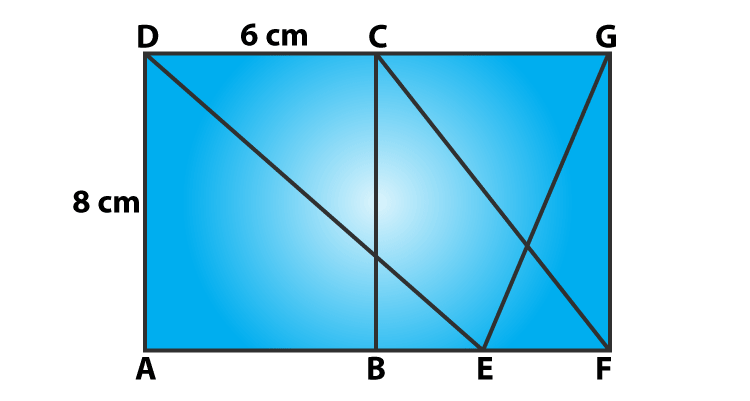Solution:

ABCD is a rectangle, where CD = 6 cm and AD = 8 cm (Given)

From figure: Parallelogram CDEF and rectangle ABCD on the same base and between the same parallels, which means both have equal areas.

Area of parallelogram CDEF = Area of rectangle ABCD ….(1)

Area of rectangle ABCD = CD x AD = 6 x 8 cm2 = 48 cm2

Equation (1) => Area of parallelogram CDEF = 48 cm2.

Question 3: In figure, find the area of ΔGEF.Solution:

From figure:

Parallelogram CDEF and rectangle ABCD on the same base and between the same parallels, which means both have equal areas.

Area of CDEF = Area of ABCD = 8 x 6 cm2= 48 cm2

Again,

Parallelogram CDEF and triangle EFG are on the same base and between the same parallels, then

Area of a triangle = ½(Area of parallelogram)

In this case,

Area of a triangle EFG = ½(Area of parallelogram CDEF) = 1/2(48 cm2) = 24 cm2.

Question 4: In figure, ABCD is a rectangle with sides AB = 10 cm and AD = 5 cm. Find the area of ΔEFG.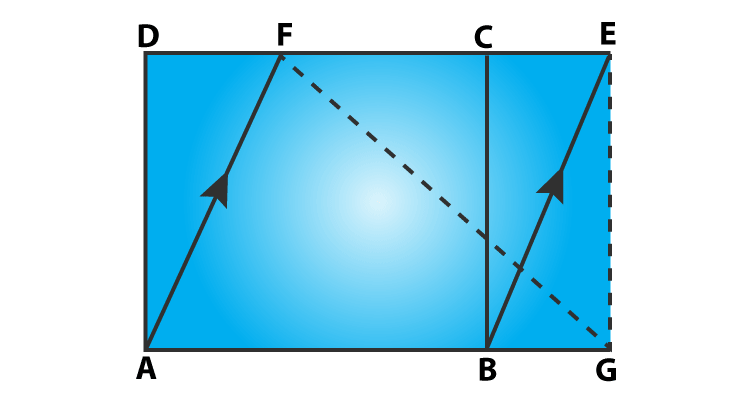Solution:

From figure:

Parallelogram ABEF and rectangle ABCD on the same base and between the same parallels, which means both have equal areas.

Area of ABEF = Area of ABCD = 10 x 5 cm2= 50 cm2

Again,

Parallelogram ABEF and triangle EFG are on the same base and between the same parallels, then

Area of a triangle = ½(Area of parallelogram)

In this case,

Area of a triangle EFG = ½(Area of parallelogram ABEF) = 1/2(50 cm2) = 25 cm2.

Question 5: PQRS is a rectangle inscribed in a quadrant of a circle of radius 13 cm. A is any point on PQ. If PS = 5 cm, then find ar(△RAS).

Solution:

PQRS is a rectangle with PS = 5 cm and PR = 13 cm (Given)

In △PSR:

Using Pythagoras theorem,

SR2 = PR2 – PS2 = (13) 2 – (5) 2 = 169 – 25 = 114

or SR = 12

Now,

Area of △RAS = 1/2 x SR x PS

= 1/2 x 12 x 5

= 30

Therefore, Area of △RAS is 30 cm2.

## RD Sharma Solutions for Class 9 Maths Chapter 15 Area of Parallelograms and Triangles Exercise VSAQs

RD Sharma Solutions Class 9 Maths Chapter 15 Area of Parallelograms and Triangles Exercise VSAQs is prepared for the revision purpose for all the concepts in chapter 15. Students are suggested to practice this exercise to check your knowledge and clear all conceptual doubts.